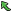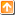## 3167: Classify Problems for Online JudgeTime Limit(Common/Java):1000MS/3000MS     Memory Limit:65536KByte
Total Submit: 6            Accepted:1

Description

Mr. Nkzgm wants to classify the problems for TZC Online Judge (). He spends a deluge of time in adding tags to every problem to represent their categories. One problem can belong to more than one category. E.g. Problem 1559 is a Graph problem, further more, it is actually a Minimum Spanning Tree problem. So Mr. Nkzgm adds ˇ®GraphˇŻ and ˇ®MinimumSpanningTreeˇŻ for the problem. Now Mr. Nkzgm decides to arrange every sort of the problems, so he needs a classifier to list all the Problem Numbers by category immediately.

Input

There is one positive integer N (N<=8999) to represent the number of the problems to be considered in the first line. In the following N lines, each line gives a Problem Number Pi (1001<=Pi<=9999, 1<=i<=N) of the problem and its categories. They are separated by a blank. A category is a string which is no more than 30 characters and consists of number, letter and underline. Line N+2 is a positive integer Q (Q<=100). In the following Q inquiry lines, each line contains one string to represent the category. There are at most 100 different categories in this problem.

Output

Please output Q lines. Each line answers the relevant inquiry. If there is any problem responding to the inquiry, the output line should start with a positive number M to represent how many problems are in this category. In the same line, after a blank, output the Problem Numbers in descending order. Each two Problem Numbers should be separated with a blank. If there is no problem in this category, the output should be ˇ®ErrorˇŻ. The inquiries contain no duplicates.

Sample Input

Sample Output

Source

NKPC4

|Back |   |Top|